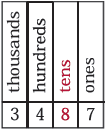# Place value

Place value is the value of a digit determined by its position in a number. In the decimal numeration system, the most commonly used numeration system, place value is based on groupings of ten and powers of ten.

 Example In the number 3,487, 8 represents 8 tens and is said to be in the tens place. Refer to the image below.### Did you know?

Computer scientists use a numeration system in which place value is based on groupings of two and powers of two. The first five numbers in this system, called the base two or binary numeration system, are 0, 1, 10, 11, and 100. Their values in base ten are 0, 1, 2, 3, and 4.

Base two has only two digits: 0 and 1. These digits are used to tell the computer what to do. 0 shows that there is no electrical current and 1 shows tha the current is on. Through the use of many 0s and 1s, computers can perform many complex operations.